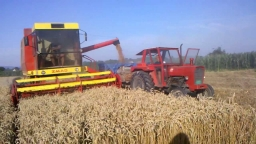# Cooperative 19973

The cooperative farmers harvested 300 tons of grain. Wheat was 25% more than oats, and rye was 96 tons less than wheat and oats combined. How many tons of wheat, rye, and oats did the cooperative harvest?

o =  88
p =  110
z =  102

### Step-by-step explanation:

p+z+o=300
p = o + 0.25o
z = p+o -96

p+z+o=300
p = o + 0.25·o
z = p+o -96

o+p+z = 300
1.25o-p = 0
o+p-z = 96

Pivot: Row 1 ↔ Row 2
1.25o-p = 0
o+p+z = 300
o+p-z = 96

Row 2 - 1/1.25 · Row 1 → Row 2
1.25o-p = 0
1.8p+z = 300
o+p-z = 96

Row 3 - 1/1.25 · Row 1 → Row 3
1.25o-p = 0
1.8p+z = 300
1.8p-z = 96

Row 3 - Row 2 → Row 3
1.25o-p = 0
1.8p+z = 300
-2z = -204

z = -204/-2 = 102
p = 300-z/1.8 = 300-102/1.8 = 110
o = 0+p/1.25 = 0+110/1.25 = 88

o = 88
p = 110
z = 102

Our linear equations calculator calculates it.Did you find an error or inaccuracy? Feel free to write us. Thank you!

Tips for related online calculators